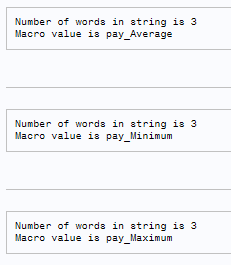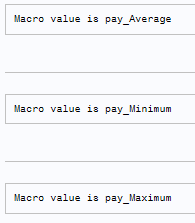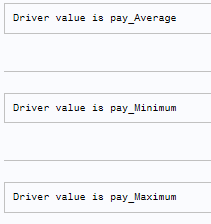## help with writing a macro to cycle through different combinations of variables

Hi, I'm very new to macro writing. Thanks in advance.  I appreciate this community.

I'd like to write a code that can cycle through combinations of:

year; max, min, and average pay (and their associated graph labels); and months of the year (and their associated graph labels)

without me having to change the combinations manually in the macro myself.

I'll paste my code and a bit of an example of the combinations below:

``````
%let Year = first;                                                                           /*looking at 10 years of data*/
%let payv=pay_Average;                                                           /*pay variable*/
%let payv =pay_Minimum;
%let payv =pay_Maximum;
%let payv _label=Average Pay ;                                              /*pay variable for figure labels*/
%let payv _label=Minimum Pay ;
%let payv _label=Maximum Pay ;
%let spendv= spend;                                                                   /*money spent variable */
%let spendv_label = money spent                                           /*money spent label for figures*/
%let months_range=BETWEEN 1 and 6;                                /*Set months range here */
%let months_range=BETWEEN 7 and 12;
%let months_label=Jan to June;                               /*Months used for figure labels*/
%let months_label=July to Dec;

``````

Three examples (out of many) of what I want the macro to do without having to manually type in the combinations:

1. The programme should output money spent for the first year of the average pay and its label on the figure should match this (i.e., payv_label is Average Pay). This will be for months 1 to 6 and its label on the figure should match this (i.e., months_label is Jan to June).
1. The programme should output money spent for the first year of minimum pay and its label on the figure should match this (i.e., payv_label is Minimum Pay). This will be for months 1 to 6 and its label on the figure should match this (i.e., months_label is Jan to June).
1. The programme should output money spent for the second year of maximum pay and its label on the figure should match this (i.e., payv_label is Maximum Pay). This will be for months 7-12 and its label on the figure should match this (i.e., months_label is July to Dec).
1 ACCEPTED SOLUTION

Accepted Solutions

## Re: help with writing a macro to cycle through different combinations of variables

SAS macros are basically SAS code generators. First step is to write static SAS code that fully works. Only once you've got such code start with the macro to make the code dynamic.

Also consider using SAS by-group processing which is much more efficient than creating many steps that each re-process the data.

You can use loops in your macro to create individual pieces of SAS code. I hope below sample code will give you the idea.

``````%macro demo(payv=);
%local n_terms;
%let n_terms=%sysfunc(countw(%str(&payv),%str( )));
%do i=1 %to &n_terms;
data _null_;
file print;
put "Number of words in string is &n_terms";
put "Macro value is %scan(&payv,&i,%str( ))";
run;
%end;
%mend;

%demo(
payv=pay_Average pay_Minimum pay_Maximum
);``````Another way often used is to have a data step that calls the macro multiple times, each time passing different parameter values. Example below.

``````%macro demo(payv=);
data _null_;
file print;
put "Macro value is &payv";
run;
%mend;

data driver;
value='pay_Average'; output;
value='pay_Minimum'; output;
value='pay_Maximum'; output;
run;

data _null_;
set driver;
length cmd \$300;
cmd=cats('%demo(payv=',value,');');
put cmd=;
call execute( cmd );
run;
``````And a 3rd option for data driven code generation is to first write the generated SAS code to a file and then %include this file for execution.

``````data driver;
value='pay_Average'; output;
value='pay_Minimum'; output;
value='pay_Maximum'; output;
run;

filename codegen temp;
data _null_;
file codegen;
set driver;
put
'data _null_;' /
'  file print;' /
'  put "Driver value is ' value '";' /
'run;'
;
run;
%include codegen /source2;``````4 REPLIES 4

## Re: help with writing a macro to cycle through different combinations of variables

The first step in writing macros is to write code that works and does what you want WITHOUT macros and WITHOUT macro variables, for one combination of variables.

Show us that code.

--
Paige Miller

## Re: help with writing a macro to cycle through different combinations of variables

This is also a lot like "please do my work for me" not a "help me with this bit I can't figure out". Which is where I start at \$100 custom programming (or more, depends).

Since you have at least 3 syntax errors in 13 lines of code things don't bode well.

Since you are discussing dates and intervals can we even hope that your current data has SAS date variables involved?

## Re: help with writing a macro to cycle through different combinations of variables

SAS macros are basically SAS code generators. First step is to write static SAS code that fully works. Only once you've got such code start with the macro to make the code dynamic.

Also consider using SAS by-group processing which is much more efficient than creating many steps that each re-process the data.

You can use loops in your macro to create individual pieces of SAS code. I hope below sample code will give you the idea.

``````%macro demo(payv=);
%local n_terms;
%let n_terms=%sysfunc(countw(%str(&payv),%str( )));
%do i=1 %to &n_terms;
data _null_;
file print;
put "Number of words in string is &n_terms";
put "Macro value is %scan(&payv,&i,%str( ))";
run;
%end;
%mend;

%demo(
payv=pay_Average pay_Minimum pay_Maximum
);``````Another way often used is to have a data step that calls the macro multiple times, each time passing different parameter values. Example below.

``````%macro demo(payv=);
data _null_;
file print;
put "Macro value is &payv";
run;
%mend;

data driver;
value='pay_Average'; output;
value='pay_Minimum'; output;
value='pay_Maximum'; output;
run;

data _null_;
set driver;
length cmd \$300;
cmd=cats('%demo(payv=',value,');');
put cmd=;
call execute( cmd );
run;
``````And a 3rd option for data driven code generation is to first write the generated SAS code to a file and then %include this file for execution.

``````data driver;
value='pay_Average'; output;
value='pay_Minimum'; output;
value='pay_Maximum'; output;
run;

filename codegen temp;
data _null_;
file codegen;
set driver;
put
'data _null_;' /
'  file print;' /
'  put "Driver value is ' value '";' /
'run;'
;
run;
%include codegen /source2;``````## Re: help with writing a macro to cycle through different combinations of variables

Thank you!

This was really helpful and I was able to make it work by referring to your first example and cobbling together some of that with what I already knew.
This is what I wrote (ignore the different variable names from my posted question- I ended up creating a dummy dataset with a simpler analysis so I could practice):

%macro a1;
%let tempvar_list=TAv TMax TMin;

/* Macro to calculate the means for specific months */
%macro calculate_means(month_var);
%do i=1 %to 3;
%let tempvar=%scan(&tempvar_list, &i);

proc means data=dat3;
where &month_var;
var &tempvar;
run;
%end;
%mend;

/* Call the macro for months 3 to 9 */
%let month_var = month >= 3 and month <= 9;
%calculate_means(&month_var);

/* Call the macro for months 5 to 8 */
%let month_var = month >= 5 and month <= 8;
%calculate_means(&month_var);

%mend;

ods listing;
%a1;
ods listing close;

Discussion stats
• 4 replies
• 239 views
• 3 likes
• 4 in conversation